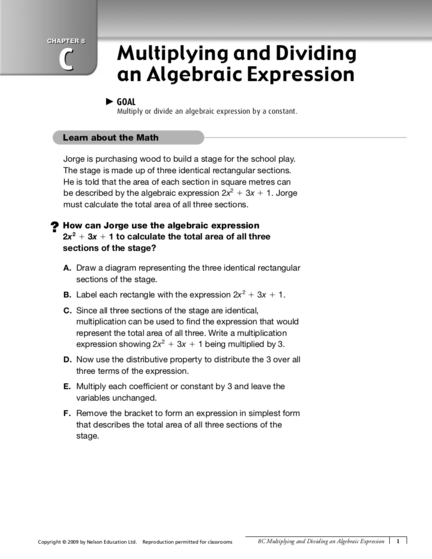# Multiplying and Dividing an Algebraic ExpressionContributed by:This pdf includes the following topics:-
Reflecting
Calculate the total area
Multiplication
Division
Examples
Solutions
1. CHAPTER 8
C Multiplying and Dividing
an Algebraic Expression
c GOAL
Multiply or divide an algebraic expression by a constant.
Jorge is purchasing wood to build a stage for the school play.
The stage is made up of three identical rectangular sections.
He is told that the area of each section in square metres can
be described by the algebraic expression 2x2 1 3x 1 1. Jorge
must calculate the total area of all three sections.
? How can Jorge use the algebraic expression
2
2x 1 3x 1 1 to calculate the total area of all three
sections of the stage?
A. Draw a diagram representing the three identical rectangular
sections of the stage.
B. Label each rectangle with the expression 2x 2 1 3x 1 1.
C. Since all three sections of the stage are identical,
multiplication can be used to find the expression that would
represent the total area of all three. Write a multiplication
expression showing 2x 2 1 3x 1 1 being multiplied by 3.
D. Now use the distributive property to distribute the 3 over all
three terms of the expression.
E. Multiply each coefficient or constant by 3 and leave the
variables unchanged.
F. Remove the bracket to form an expression in simplest form
that describes the total area of all three sections of the
stage.
Copyright © 2009 by Nelson Education Ltd. Reproduction permitted for classrooms 8C Multiplying and Dividing an Algebraic Expression 1
2. G. Jorge is told that if he replaces the x terms by 1, he will
be able to calculate the actual area of the stage as a
numerical value. Substitute 1 into your expression for x.
H. Simplify the expression. The solution will be equal to the
total area of the three sections of the stage, in square
metres.
Reflecting
1. If an expression has three terms, how many terms will it
have after being multiplied by a constant? Explain.
2. If instead of 3, you were to multiply the expression by 23,
3. How would you expect dividing an expression by a
constant to differ from multiplying it by a constant?
Work with the Math
Example 1: Multiplying an algebraic expression by a constant
Multiply 25(2x3 1 6x2 2 8x).
Akeem’s Solution
(25 3 2x 3 ) 1 (25 3 6x 2 ) 2 (25 3 8x) First, I must use the distributive
property to distribute the 25 over
all three terms in this expression.
(210x3 ) 1 (230x2 ) 2 (240x) Then, I multiply each coefficient by
25 and leave the variables
unchanged.
210x3 2 30x2 1 40x Finally, I remove the bracket and
write in simplest form.
25(2x3 1 6x2 2 8x) 5 210x3 2 30x2 1 40x
2 Nelson Mathematics Secondary Year Two, Cycle One Reproduction permitted for classrooms Copyright © 2009 by Nelson Education Ltd.
3. Example 2: Dividing an algebraic expression by a constant
Divide (10x3 1 12x2 2 6x 1 2) by 2.
Denise’s Solution
10 3 12 2 6 2
x 1 x 2 x1 I must rewrite the expression, dividing every
2 2 2 2
coefficient or constant by the divisor, 2.
5x 3 1 6x 2 2 3x 1 1 Then, I use division to simplify the coefficients
and constants and leave the variables unchanged.
(10x3 1 12x2 2 6x 1 2) 4 2 5 5x3 1 6x2 2 3x 1 1
A Checking C Extending
2
4. Multiply 10(3x 1 7x 1 7). 8. Write each expression in simplest
5. Divide (9x3 1 18x2 1 12x) by 3. form.
B Practising a) 2(10x2 1 15) 4 10
6. Distribute the constant over each term
b) 25(12x4 1 7x3 1 28x2 1 24x) 4 5
in the following expressions.
c) 7(x2 1 11 1 8x) 1 3(x2 2 4)
d) 3(3x2 1 6x 2 9) 4 9
a) 8(12x2 2 3) e) 2(2(2(x 1 1))) 4 8
b) 11(14x3 1 8x 1 5) f) 2(x 2 1 1) 2 3(x 1 1)
c) 220(10x2 2 9x 1 22)
9. A rectangle has dimensions 3 m by
d) 15(x4 1 5x3 2 15x2 1 x 2 1)
2x2 1 4x 1 8 metres. What is the area
e) 24(100x2 1 44x 1 5)
of each of the triangles formed by
7. Find each product or quotient. Write drawing one diagonal of the rectangle?
10. If c and d are whole numbers
a) 12(3x2 1 6x 2 4) and neither c nor d is 0, what
b) 27(11x3 2 2x 1 18) must be true about c and d if the
c) (12x2 1 4x 1 16) 4 4 coefficients of c(2x2 1 3x 1 1) 4 d
d) 24(22x4 2 3x3 1 4x2 1 2x 2 20) are whole numbers?
e) (25x2 1 10x 2 5) 4 (25)
f) 20(10x3 2 5x 1 11)
g) (35x2 1 28x 2 14) 4 7
h) (54x4 2 36x3 2 27x2 1 9x 2 18) 4 9
i) (62x2 1 2x 2 4) 4 (22)
j) 28(5x4 1 16x2 1 8x 2 1)
k) 88(10x2 2 9x)
l) (50x2 2 30x 1 40) 4 10
m) (121x2 1 11x) 4 11
Copyright © 2009 by Nelson Education Ltd. Reproduction permitted for classrooms 8C Multiplying and Dividing an Algebraic Expression 3